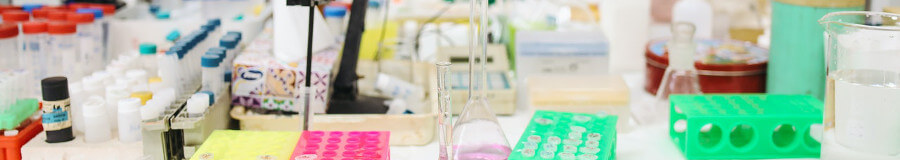When choosing a lab balance and evaluating whether it fits your criteria, uncertainty is not as well-known as other factors such as readability or linearity, but it’s just as important. While uncertainty is used in a wide variety of sciences and variables, in this blog post, we’ll help you understand weighing uncertainty as it pertains to laboratory balances.

### What is Weighing Uncertainty?

Weighing uncertainty is the range of values that can be reasonably assumed to be within the measurement’s exact value. It’s a clunky definition, so let’s unpack it.

For example, when you measure powder and the balance tells you it weighs 0.0067g, you assume that the mass of the powder is 0.0067g. Depending on the balance’s readability, you could assume it was rounded up or down (so it could really be 0.006712g or 0.006699g, as your balance only displays 4 digits after the decimal) but that your results are still within an acceptable range of the true value of the measurement.

Laboratories set requirements known as tolerances to account for uncertainty. As long as a balance’s uncertainty falls within the tolerances set by the guidelines, it is acceptable.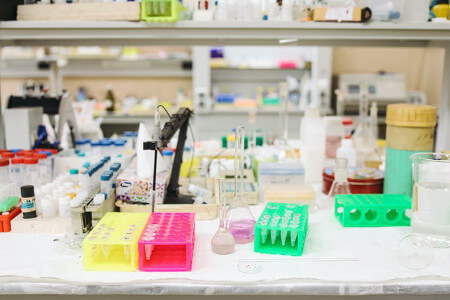### What is the Difference Between Accuracy and Uncertainty?

We define accuracy as “the ability to display a value that matches the ideal value for a known weight.” If that sounds a lot like the definition for uncertainty, you’re not alone in making that mistake. The two terms are often used interchangeably because of their relationship, despite being two distinct concepts. Accuracy indicates how close a reading is to the exact value, while uncertainty deals with factors that could affect the result’s accuracy. So, accuracy tells you how similar the measured value and the exact value are, and uncertainty quantifies that similarity to let you know how reliable it is. Still scratching your head? Let’s use an example.

Let’s say you’re measuring a sample. You measure it on a balance a few times (only for the sake of the example; such a test should be done at least ten times in a laboratory setting), and you get 0.0100g, 0.0101g, 0.0102g and 0.0100g. This means your result range is between 0.0100g and 0.0102g. Depending on your balance’s class and your lab’s requirements, you can check accuracy by making the weigh tested falls within a certain percentage of the true value (0.10% is often used, but it might depend on local metrology and your own laboratory). But how certain are you that the weight is actually within that range? That’s where uncertainty comes in. By calculating uncertainty, you can get a number (usually a percentage) expressing just how sure you can be that the measurement truly falls between that range.

### Why is Uncertainty an Important Parameter for Measurements?

Uncertainty is a key factor in understanding and analyzing an instrument’s calibration results, sensitivity, readability, repeatability, standard deviation and more. It must be properly calculated for quality control measures, as it’s one of the variables that can dictate if a product or test is acceptable.

It’s impossible to obtain laboratory accreditation without proper uncertainty measurements. Calculating uncertainty allows users to set tolerances that ensure repeatable results with high standards. For a lab that measures very small substances that can be dangerous if present in high enough concentration, uncertainty measurements are essential, as they can help provide reliable results. Uncertainty is fundamental for calibration laboratories because they set the standard by which all results will be judged against.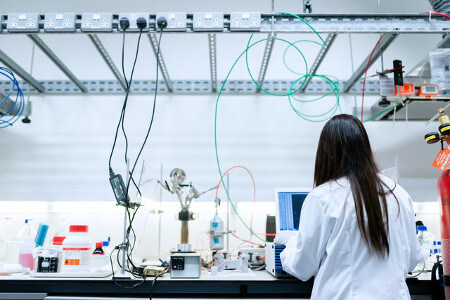In addition, uncertainty can be used to judge an experiment’s quality and allows people to compare results more meaningfully. If a laboratory tells you they made twenty measurements and got results that were precise and accurate, you might be impressed with their results. But let’s say they’re only 67% sure that their results are accurate and 69% sure that they’re precise. Is that still impressive?

It also helps people compare experiments. If two teams conduct experiments to prove that a substance has a certain effect on people, their uncertainty measurement is a good way to see which team created the better experiment and if they can even be compared.

### What’s the Relationship Between Uncertainty and Standard Deviation?

If you thought the difference between accuracy and uncertainty was confusing, I’m sorry to say standard deviation is not going to help. Standard deviation is the difference between the measured values and the true values. Accuracy is how close these values are, and uncertainty is how reliable the measured values are. Standard deviation is a factor that affects uncertainty. There is a direct correlation: the higher the standard deviation, the higher the uncertainty (and vice versa). Uncertainty relies on other factors as well, but standard deviation’s effect is highly noticeable and tends to be a good parameter to evaluate uncertainty.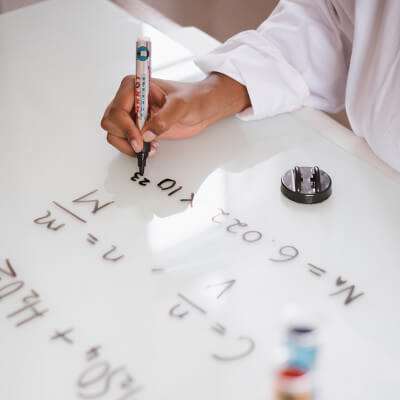Standard deviation affects many different variables, but one of the most useful calculations is to determine the standard deviation of repeatability.

1. To do so, make multiple measurements with the same balance and sample under the same conditions (including user and environmental conditions) and record each result.
2. Calculate the mean by adding all the results together and dividing the sum of the results by the number of results.
3. Subtract each result from the mean, then square the difference. You should have only positive numbers at this stage.
4. Add the squared results together.
5. Divide the sum of the squared results by the number of results minus one.
6. Get the square root of the quotient.
7. (Optional) If you want the standard deviation of the mean, take the last result and divide it by the square root of the number of results.
8. To get the standard deviation as a percentage (relative standard deviation), multiply the standard deviation by 100, then divide the product by the mean.

Example:

1. Four measurements: 2, 3 ,2, 2
2. (2+3+2+2) / 4 = (9) / 4 = 2.25
3. 2.25 – 2 = 0.25. 2.25 – 3 = -0.75. 2.25 – 2 = 0.25. 2.25 – 2 = 0.25. 2.252 = 0.0625. -0.752 = 0.5625. 2.252 = 0.0625. 2.252 = 0.0625.
4. 0.0625 + 0.5625 + 0.0625 + 0.0625 = 0.75
5. 0.75 / (4 – 1) = 0.75 / 3 = 0.25
6. √0.25 = 0.5. Rstd = 0.5.
7. 0.5 / √4 = 0.5 / 2 = 0.25. RstdM = 0.25.
8. (0.5 * 100) / 2.25 = 22.22%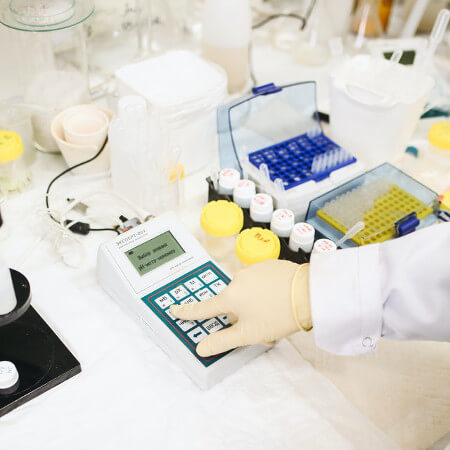### Uncertainty and Minimum Weight

If you’ve browsed specifications for laboratory balances, you might have noticed the minimum weight. The minimum weight is the smallest quantity of matter that can be measured on an instrument without its uncertainty going over the tolerance required. That is not the same thing as the smallest amount of weight a balance can measure, merely how small the weight can be before the results are considered too unreliable to be used for certain applications.

A balance can measure an amount smaller than the minimum weight, but with reduced certainty. Minimum weight is not a stable value because of various factors that affect uncertainty and standard deviation, which is why is it expressed with ±.

Most laboratories recommend that you always weigh above the minimum weight to ensure reliable results. That means if you need to measure small quantities reliably, you should pay close attention to a balance’s minimum weight and if it fits within your laboratory’s tolerances. This is why some laboratories seem to use balances more precise than they need; while an analytical balance might have readability that goes high enough, a scientist might need the minimum weight of a semi-micro balance instead.

### How does Uncertainty Differ from Errors?

While they are often used interchangeably, uncertainty is not the same as an error. While errors can affect uncertainty, they are mistakes that cause some kind of malfunction and prevents the balance from providing correct results. They can increase uncertainty, but their tolerances should be much, much lower.

Uncertainty is unavoidable because of the sheer nature of measurements: no matter how something is made, there is a chance, however slight, that there could be some deviance between the expected mass and the final mass. It is not a mistake, merely a biproduct of the world we live in, and can be influenced by many different factors.

Essentially, uncertainty means that no matter how precise and accurate a balance is, and no matter how a product should weigh, there might be slight variance because we do not know the exact weight, and even if we do, outside factors may have changed it. An error is a problem that causes the balance to give the wrong reading.### How Do You Calculate the Uncertainty of a Lab Balance?

Uncertainty is not a number that is associated with a single reading. Rather, it’s a measure of how reliable results are. That means that when uncertainty is calculated, it needs to be calculated for readability, repeatability, calibration, density, reference mass, error, weighing result, environmental influences and many, many more factors. There is sadly no clear cut answer, since uncertainty needs to be measured for each value that needs to be evaluated. These uncertainty results then need to be combined in equations that account for their individual importance and which readings affect each other. The result of each calculation needs to be looked at as a full set to truly get the full picture of a balance’s reliability. Because of the complexity associated with calculating uncertainty, it’s often done by calibration laboratories who have the knowledge required to properly conduct a multitude of complex testing procedures.

### What is an Acceptable Percentage of Weighing Uncertainty?

That really depends on the laboratory and the types of samples you need to measure. A pharmaceutical laboratory and a calibration laboratory could have different acceptable percentages, depending on the balance and samples. Because it’s so specific to the application, you must refer to your laboratory’s guidelines and tolerances.

Generally, uncertainty can be expressed as the sample’s weight (the value of measured quantity), the ± sign and the value of the measurement uncertainty itself. So if a balance has an uncertainty measurement of 1mg and you’re measuring 10g, the result should be 10±0.01%. Ideally, while the number should be as low as possible. How low really depends on your tolerances.

Weighing uncertainty is a complex topic that is necessary to understand when looking at lab balances. It affects almost all facets of weight measurements. If you have questions about weighing uncertainty or need help finding the right lab balance, please reach out! We’ll be happy to help you.